# Length and AreaPage 2

#### WATCH ALL SLIDES

= ½ × b × h

Slide 11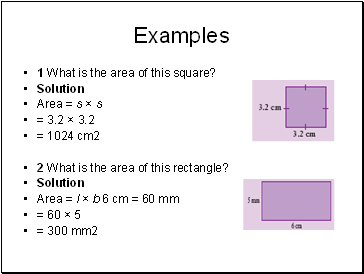Examples

1 What is the area of this square?

Solution

Area = s × s

= 3.2 × 3.2

= 1024 cm2

2 What is the area of this rectangle?

Solution

Area = l × b 6 cm = 60 mm

= 60 × 5

= 300 mm2

Slide 12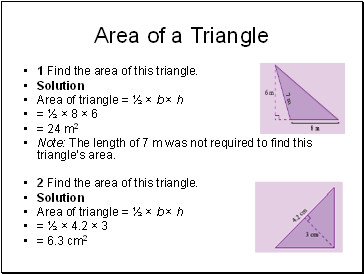## Area of a Triangle

1 Find the area of this triangle.

Solution

Area of triangle = ½ × b × h

= ½ × 8 × 6

= 24 m2

Note: The length of 7 m was not required to find this triangle’s area.

2 Find the area of this triangle.

Solution

Area of triangle = ½ × b × h

= ½ × 4.2 × 3

= 6.3 cm2

Slide 13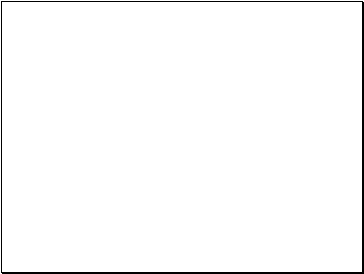Slide 14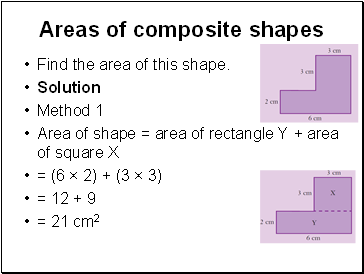## Areas of composite shapes

Find the area of this shape.

Solution

Method 1

Area of shape = area of rectangle Y + area of square X

= (6 × 2) + (3 × 3)

= 12 + 9

= 21 cm2

Slide 15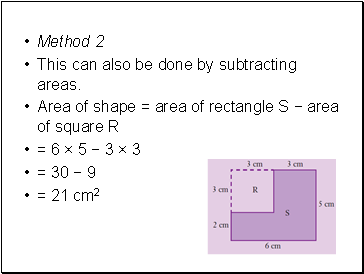Method 2

This can also be done by subtracting areas.

Area of shape = area of rectangle S − area of square R

= 6 × 5 − 3 × 3

= 30 − 9

= 21 cm2

Slide 16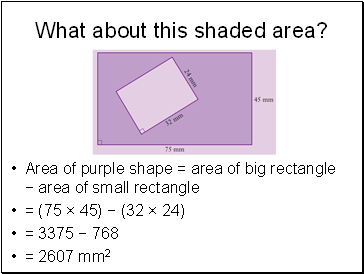Area of purple shape = area of big rectangle − area of small rectangle

= (75 × 45) − (32 × 24)

= 3375 − 768

= 2607 mm2

Slide 17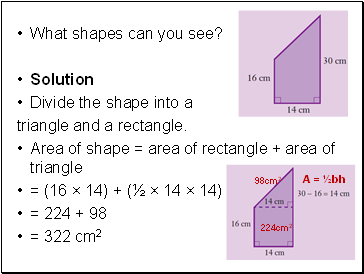What shapes can you see?

Solution

Divide the shape into a

triangle and a rectangle.

Area of shape = area of rectangle + area of triangle

= (16 × 14) + (½ × 14 × 14)

= 224 + 98

= 322 cm2

224cm2

A = ½bh

98cm2

Slide 18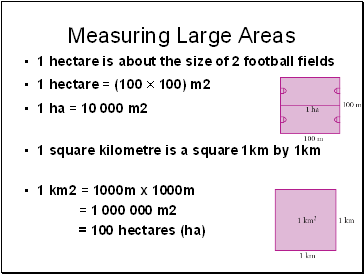## Measuring Large Areas

1 hectare is about the size of 2 football fields

1 hectare = (100 × 100) m2

1 ha = 10 000 m2

1 square kilometre is a square 1km by 1km

1 km2 = 1000m x 1000m

= 1 000 000 m2

= 100 hectares (ha)

Slide 19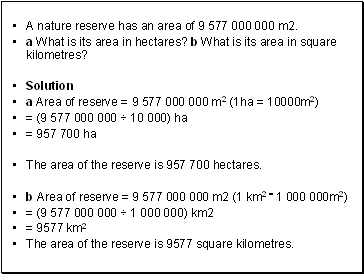A nature reserve has an area of 9 577 000 000 m2.

a What is its area in hectares? b What is its area in square kilometres?

Solution

a Area of reserve = 9 577 000 000 m2 (1ha = 10000m2)

= (9 577 000 000 ÷ 10 000) ha

Go to page:
1  2  3